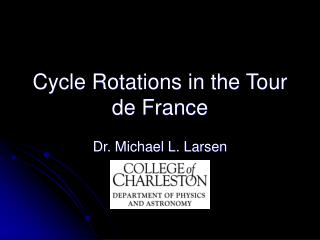# Cycle Rotations in the Tour de France - PowerPoint PPT PresentationDownload PresentationCycle Rotations in the Tour de France

Cycle Rotations in the Tour de FranceDownload Presentation## Cycle Rotations in the Tour de France

- - - - - - - - - - - - - - - - - - - - - - - - - - - E N D - - - - - - - - - - - - - - - - - - - - - - - - - - -
##### Presentation Transcript

1. Cycle Rotations in the Tour de France Dr. Michael L. Larsen

2. Relevant Background • Tour de France is a “tour of France” • Held annually since 1903 (except for during WWI and WWII) • Length varies by year. • Shortest = 2420 km • Longest = 5745 km • 2013 = 3360 km (about average)

3. More Relevant Background • Race run in “stages” (21) • 3 Time trials (very brief – 20-50 km) • Other stages. • 2013 details: • 7 flat stages • 5 hilly stages • 6 mountain stages

4. 2013 Route

5. The Question: “How many times do the pedals turn for the Tour-de-France winner”

6. Methodology • 2 Approaches • Approach 1: • Use the distance of the race, parameters of the bike, gear ratios, and wheel sizes to reason out how many times the pedals turned. • Approach 2: • Use the winning total time and videos of racers to determine how many rotations per second. Multiply the two to estimate answer.

7. Distance Based Approach • Total distance = 3360 km • Wheel size: 650-700 mm diameter • Gears: • High gear = 53 tooth chain on 11 tooth gear. • Low gear = 39 tooth chain on 25 tooth sprocket.

8. Distance per rotation • Largest possible: • (53/11) x (pi) x (0.7m) = 10.6 meters/rotation • Smallest possible: • (39/25) x (pi) x (0.65m) = 3.2 meters/rotation

9. Method One – Crude Bounds • Total distance is 3360 km = 3.36 x 106 meters. • Maximum total turns = (3.36 x 106 m) / (3.2 meters/turn) = 1.05 million turns • Minimum total turns = (3.36 x 106 m) / (10.6 meters/turn) = 317,000 turns

10. Best estimates • Assume use something close to highest gear on flat ground and something close to lowest gear on Mountain stages. (Very approximate). • Downhill slopes? • Not perfectly flat/sprinting areas? • Divide into two pieces; calculate amount of turns on each piece and add them up.

11. Mountain Stages 2013 • Stage 8 (176 km) • Stage 9 (165 km) • Stage 15 (242 km) • Stage 18 (168 km) • Stage 19 (204 km) • Stage 20 (125 km) Total mountain stages: 1080 km Total non-mountain: 2280 km

12. Refined Estimate • 2280 km (flat) x (1000 m/km) x (1 rotation/9m) = 253,000 rotations • 1080 km (mountain) x (1000 m/km) x (1 rotation/5m) = 216,000 rotations • Total = 253,000+216,000 = 469,000 ~ ½ a million rotations

13. Method 2: Time-Based • Winners typically finish in about 90 hours of real cycling time. • Youtube videos suggest that most cyclists rotate about 1.1 cycles/second during climbs and about 1.5 cycles/second during flat parts.

14. Time-Based Estimate • 90 hours x (3600 sec/hour) = 324,000 sec • 324,000 sec x (1.35 rot/sec) = 437,000 rotations total

15. Summary • Based on distances, wheel sizes, and gear ratios we came up with a lower-bound of 320,000 rotations and an upper bound of 1.05 million rotations. • A more precise estimate based on distances, wheel sizes, and gear ratios gave us a value of about 500,000 rotations. • Based on time, we estimate 437,000 rotations

16. Major Possible Errors or Erroneous Assumptions • Crude estimation on breakdown of gear by stage type. • Ultimate Impact on Result – Either Direction • Assumed constant pedaling (no coasting) in distance-based calculation. • Ultimate Impact on Result – Overestimate • Assumed pedaling at all times in time-based calculation. • Ultimate Impact on Result – Overestimate

17. Final Answer • We are certain the final answer lies somewhere between 250k rotations and 1M rotations. • Using our two methods, we found a value between 400k and 500k rotations, though most of the errors in these methods both result in overestimation. • Consequently, we believe the answer is likely in the range 300,000 < X < 500,000 and certainly in the range 250,000 < X < 1,000,000

18. Refinements • Extra distance travelled due to moving around other bikers (assumed negligible). • More sophisticated/detailed treatment of gear ratios based on terrain. (Current treatment crudely handles downhill sections) • Better estimate of pedaling rate based on more video measurements.

19. Thank You For Your Attention • Any questions?